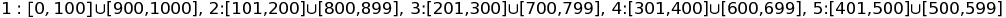# Parallel Ramanujan's formula for 1/π calculation

I finished my university project for calculating $$\1/\pi\$$ and I would love to get some feedback.

Before you guys jump into this code please keep in mind newcomer to C++ just decided to use it for this project because I assumed libraries will be better than others in terms of arbitrary precision.

Few words about the project, it should be a console application with some parameters like (how many terms of the formula to be calculated, how many threads should be used, log level and more if desired).

I am using MPFR and MPIR on Windows for the arbitrary precision calculations and Visual C++ compiler with no extra flags other than the one set up by Visual Studio (perhaps I can be advised here :) ).

I am interested in following feedbacks priority is in the given order

1. Performance
3. Design?

Since the project tends to be big I will only put the parts I am interested the most (calculation) if anyone else would like to advise me over the overall design please check my repository for the full code base.

main.cpp

boost::multiprecision::mpfr_float inc_multiply(long start, long end)
{
boost::multiprecision::mpfr_float fact = 1;
for (; start <= end; ++start)
fact *= start;

return fact;
}
// <-- the real calculation of pi
boost::multiprecision::mpfr_float calculatePi(int count, ...)
{
using boost::multiprecision::mpfr_float;
mpfr_float partition;

std::stringstream ss;

auto clock_start = std::chrono::system_clock::now();

std::va_list ranges;
va_start(ranges, count);

for (int i = 0; i < count; ++i)
{
auto boundary = va_arg(ranges, range_t);
mpfr_float previous_fac_start = 1.0;
mpfr_float previous_fac_4xstart = 1.0;

for (; boundary.first < boundary.second; ++boundary.first)
{
mpfr_float fac_start = previous_fac_start * (boundary.first == 0 ? 1 : boundary.first);
mpfr_float fac_4xstart = previous_fac_4xstart * inc_multiply((4 * boundary.first - 3) < 0 ? 1 : 4 * boundary.first - 3, 4 * boundary.first);

mpfr_float n = fac_4xstart * mpfr_float(1103 + 26390 * boundary.first);
mpfr_float d = boost::multiprecision::pow(fac_start, 4) * boost::multiprecision::pow((mpfr_float)396, 4 * boundary.first);

partition += (n / d);
previous_fac_start = fac_start;
previous_fac_4xstart = fac_4xstart;
}
}

va_end(ranges);

std::chrono::duration<double> elapsed = std::chrono::system_clock::now() - clock_start;
LOG_VERBOSE("Thread " + thread_id + " execution time was " + std::to_string(elapsed.count()) + "ms\n");

return (2 * boost::multiprecision::sqrt((mpfr_float)2) / 9801) * partition;
}
// <-- used to setup the strategy for calculation
boost::multiprecision::mpfr_float calculate(ProgramOptions* options)
{
std::vector<std::future<boost::multiprecision::mpfr_float>> futures;

// Choose thread work sepration strategy
// TODO: Fix duplication, think of dynamic creation of strategies.
if (options->IsOptimized())
{
auto strategy = new OptimizedSeparationStrategy();

for (auto partition : partitions)
futures.emplace_back(std::async(calculatePi, 2, partition.first, partition.second));
}
else
{
auto strategy = new EqualSeparationStrategy();

for (auto partition : partitions)
futures.emplace_back(std::async(calculatePi, 1, partition));
}

boost::multiprecision::mpfr_float pi;

return pi;
}


SeparationStrategy.cpp

simple_ranges NaiveSeparationStrategy::Separate(long iterations, short threads)
{
simple_ranges ranges;
return ranges;

auto chunk = iterations / threads;
auto remainder = iterations % threads;

auto loopIterations = 0;
for (auto partition = 0; partition <= iterations - chunk + loopIterations; partition += chunk + 1)
{
ranges.emplace_back(partition, partition + chunk > iterations ? iterations : partition + chunk);
++loopIterations;
}

return ranges;
}

{
return ranges;

auto leftChunk = 0, rightChunk = 0;

if ((iterations / threads) % 2 != 0)
{
leftChunk = (iterations / threads) / 2;
rightChunk = (iterations / threads) / 2 + 1;
}
else
{
leftChunk = rightChunk = (iterations / threads) / 2;
}

long l = 0, r = iterations, previous_l = l, previous_r = r;

while (l < r)
{
ranges.emplace_back(std::make_pair(previous_l, l + leftChunk), std::make_pair(r - rightChunk, previous_r));

previous_l = l + leftChunk + 1;
previous_r = r - rightChunk - 1;

l += leftChunk;
r -= rightChunk;
}

// make last range excluding last number
// as it will be calculated from the second last range.
ranges.back().first.second--;

return ranges;
}


Couple of things I found myself so far.

### Performance wise

Huge mistake, to use std::async for multiprocessing, while testing it on my local machine with only one CPU it worked okay, using all the cores, but when switched to test server with more than one CPU, I failed to use all of them (actually 32). Changing this std::thread , without any pain btw, did the job and I wasable to utilize all the CPUs on the test server.

### Design

My so-called "optimized" separation of work of the threads prevents me from implementing graceful shutdown eventually because if I need to calculate 1000 terms of this formula, work is separated like sowhere 1,2,3... are thread_ids. A separation like this is good for maintaining equal work among your threads, but like I said it prevents the ability to provide graceful shutdown due to calculation not being done in a serial matter.

Well, so far, this is what I've found, I will add more to the list whenever I found other problems with my code.